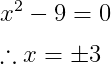# How to represent therefore symbol(∴) in LaTeX?

“Therefore” is written in the form of words and expressed in the form of symbol.

Mathematically, this symbol is represented by ∴ . And to represent this therefore symbol in latex, you need to use the \therefore command.

Properties Value
Symbol Therefore
Package amssymb
Command \therefore
Argument No
Example \therefore → ∴

And \therefore commands are not available by default in latex. For this, you need to install amssymb package.

For example, you may have noticed that in most cases this therefore symbol is used to determine the final value of the equation.

\documentclass{article}
\usepackage{amssymb}
\begin{document}
$$\\x^{2}-9=0$$
$$\therefore x=\pm 3$$
\end{document}

Output :You can see the latex code above. Here \usepackage command is used to install the external package.#### Md Jidan Mondal

LaTeX expert with over 10 years of experience in document preparation and typesetting. Specializes in creating professional documents, reports, and presentations using LaTeX.